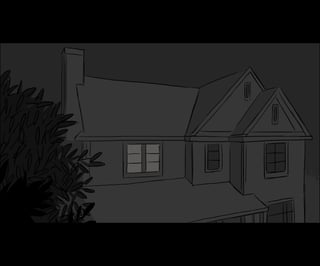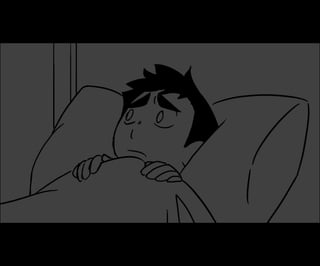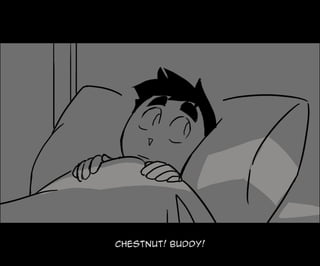Diese Präsentation wurde erfolgreich gemeldet.

# Autophobia- Parasomnia round 1 pt 1

Anzeige
Anzeige
Anzeige
Anzeige
Anzeige
Anzeige
Anzeige
Anzeige
Anzeige
Anzeige
Anzeige×

## Hier ansehen

1 von 215 Anzeige

# Autophobia- Parasomnia round 1 pt 1

Apo and Peri who belong to @dmyymo @Armigegress
part 2: https://www.slideshare.net/Bluefrknsky/autophobia-parasomnia-round-1-pt-2

Apo and Peri who belong to @dmyymo @Armigegress
part 2: https://www.slideshare.net/Bluefrknsky/autophobia-parasomnia-round-1-pt-2

Anzeige
Anzeige

### Autophobia- Parasomnia round 1 pt 1

1. 1. c h e st nu t !b u ddy !
2. 2. st i l lawak e ?
3. 3. Ah , oneoft h oseni gh t s?
4. 4. Ik now wh at ’ l lge tt h ose b ad v i b e s ou t t a y ou r h e ad:
5. 5. oneofmy sp e c i alb e dt i mest or i e s!
6. 6. I ’ l lgi v ey a a good one
7. 7. ab ou th e r oe s and a c au l dr on
8. 8. t h atc ou l d b r i ng b ac kt h ede ad!
9. 9. as gr anp ap i wou l d say , “ Amse r Mai t hy n ol ” . . .
10. 10. “ onc eu p on a t i me ” . . .
11. 11. i al r e ady t ol d y ou , i don’ tk now!
12. 12. some t h i ng p u l l e d mei nt o t h ee l e v at or , e v e r y t h i ng we ntb l ac k . . .
13. 13. . . . and wh e n i wok eu pi was some wh e r e e l sei n t h eb u i l di ng
14. 14. H one st l y , Idon’ tk now h ow sh i t l i k et h i s k e e p s h ap p e ni ng
15. 15. C au sey ou ne v e r c h ooset o do t h ese nsi b l et h i ng
16. 16. and ju stt r u stwh at ’ s gi v e n t o y ou .
17. 17. Don, i t ’ s notl i k eh ewou l d k now t h i s was goi ng t o h ap p e n
18. 18. i fwemay , p e r h ap s, go b ac kt o t h et op i c . . .
19. 19. Wec ou l dn’ tt r ac eany c omp any c al l e d P ar asomni a ar ou nd t h ear e a.
20. 20. Or any wh e r ef or t h atmat t e r , so wh oe v e r k i dnap p e d y ou l i e d t o y ou r f ac e
21. 21. and y ou ’ r ei n someab andone d l otsome wh e r e .
22. 22. i tdoe sn’ tl ookab andone d, t h at ’ s f or su r e . . . ju ste mp t y
23. 23. wh i c hme ans we ’ r egonna h av et o f i nd ou tmor e i fIwanna ge tou t t a t h i s h e l l h ol e
24. 24. may b ei t ’ s ju sta f r ontb u si ne ss. . .
25. 25. e v e n f r ontb u si ne sse s h av el i c e nse s- -
26. 26. P l e aseb esaf e , C h e s.
27. 27. { b e e p}
28. 28. H e y !
29. 29. H e y , i s any oneou tt h e r e ? !I ’ m c age d down h e r e .
30. 30. Y ou me an l i k ei n t h ev e nti t se l f ?
31. 31. Nott h egotdang v e nt , Ime an down i n t h i s p r i son t h e y gotmei n!
32. 32. P r i son? Wh e r et h ef u c kwou l d t h ate v e n b ei n an of f i c eb u i l di ng?
33. 33. Ic an’ tse ea dar ne d t h i ng b u tImanage d t o se esome t h i n’ l i k emoni t or s, i tl ook s l i k ea b ase me nt .
34. 34. B ase me ntwi t hmoni t or s…
35. 35. I ’ l lse ei fIc an t r y and f i nd y ou - -
36. 36. c an’ tb et h atf ar , b u t . . . i se eno moni t or s. . .
37. 37. S E C U R I T Y
38. 38. u h h , h e l l o?
39. 39. Y ou ’ r eh e r e !T h ankt h eh e av e ns. Now c omeand h e l pmege tt h eh e l lou t t a t h i s b ox .
40. 40. Y ou ’ r eh e r e !T h ankt h eh e av e ns. Now c omeand h e l pmege tt h eh e l lou t t a t h i s b ox .
41. 41. { b e e p}
42. 42. Ah !t h e r ewego!
43. 43. T h anky a k i ndl y f or ge t t i ng meou tt h e r e , If e e l l i k eIwas gonna go st i r c r az y i n t h atl i l ’ r oom.
44. 44. mi nd h andi ng memy h amme r ?
45. 45. wh ate v e n goty ou r st u c ki n h e r e ?
46. 46. h e c ki fi k now!wok eu pi n t h i s b u i l di ng and b e f or ei k ne w i tt h e y t ookou r we ap ons
47. 47. good t o k now i ’ m nott h eonl y one dr agge d i nt o t h i s p l ac e . . .
48. 48. I fy ou h av ea K e y , t h e n y ou h av et h eway ou t .
49. 49. T ak emet h e r eor I ’ l lsp i l ly ou r gu t s h e r eand now t h e n MAK Ey ou .
50. 50. Wh att h ef U C K ?
51. 51. L i k eh e l lIam!
52. 52. F owlmou t h e d and u nwi se . Y ou f i twe l lwi t ht h ef i l t ht h ati s wi t hy ou …
53. 53. F owlmou t h e d and u nwi se . Y ou f i twe l lwi t ht h ef i l t ht h ati s wi t hy ou …
54. 54. h ol y sh i t !
55. 55. wh at ’ s y ou r f r i e nd’ s p r ob l e m? !
56. 56. Ne v e r sai d Iwas f r i e nds wi t ht h edamne d b ou nt y !
57. 57. B OU NT Y ? !
58. 58. E asi l y di st r ac t e d as we l las st u p i d.
59. 59. T h at ’ s i t !
60. 60. T h atwas c l ose .
61. 61. H ow l ong ar et h e seb i nds goi ng t o h ol d any way s?
62. 62. As l ong as Iwantt h e m t o b u c k o. t h osear e n’ tf l i msy h e mp e n af t e r al l .
63. 63. h u p .
64. 64. Wh atnow t h ou gh ?
65. 65. Wh adda y a me an, wh atnow? wef i nd h ow t o ge tou t t a h e r e , c ou r se .
66. 66. Wh e n Idi se nt angl ef r om t h e seb i nds, I ’ l lf l ay y ou b ot hal i v e .
67. 67. Iwi l ll e ty ou r e l i shi n y ou r own mi st ak e s f or st op p i ng me
68. 68. t h e n onc ey ou c ol l ap set o t h e f l oor , gi v i ng y ou r l astb r e at h s ofl i f e
69. 69. Iwi l lr ai sey ou r b odi e s so y ou k now no p e ac ewi t h i n de at h .
70. 70. C H r i st , y ou ’ r ea dar k - mi nde d l i t t l ef u c kar e n’ ty ou ?
71. 71. Ange r i s nott h ewor d. I ti s annoy anc e .
72. 72. y ou k now, i c an’ th e l pb u tb er e mi nde d ofanot h e r e dgy b ast ar d l i k ey ou .
73. 73. b y t h eb y , If or gott o ask .
74. 74. wh at ’ s y ou r name ?
75. 75. u h h , r i gh t . . . i t ’ s c h e st e r . . .
76. 76. We l l , C h e st e r , y a c an c al lmeap o!
77. 77. . . . Wh e r ear ey ou f r om?
78. 78. e h , Iju stf ol l ow wh e r e v e r t h ef r e e dom f i r e s go.
79. 79. Man, t h i s p l ac ei s t h ewh ol ek i t and k ab oodl eofc r e e p sh ow, Ai n’ tno
80. 80. oneh e r eand ai n’ tno noi se c e p tt h eh u mmi n ofsomet e c h .
81. 81. Wai ta se c ond. . . wh ati f . . . Wh ati fmy mi nd madet h e m u p ? !
82. 82. T h i s onei s a l otl i k ea b i z ar r ec ar t oon v e r si on ofu nc l ewi n. . .
83. 83. and t h eot h e r i s l i k ea me sse d u pl awr e nc e . . .
84. 84. wh ati ft h i s p l ac ei s me ssi ng u pwi t hmy h e ad? !
85. 85. h e y u h h , ap o, r i gh t . . . ?
86. 86. ar ey ou r e al ?
87. 87. { k i c k}
88. 88. ow!mot h e r f - -
89. 89. Doe s t h atf e e lr e al ?
90. 90. Igu e ss!Sh i t - -
91. 91. Atl e astIk now y ou ’ r enotsome t wi st e d v e r si on ofh i m… H e ’ d ne v e r do t h at .
92. 92. Wh o?
93. 93. some oneIk now- - y ou k i nda. . . sou nd l i k ee m.
94. 94. So y ou c ameh e r eaf t e r some one , h u h .
95. 95. Wh atdoe s h el ookl i k e ?
96. 96. U h hab ou tmy h e i gh t . . . b r own h ai r , f r e c k l e s, mu l l e t . . . al way s we ar s a p ai r ofgoggl e s on h i s f or e h e ad
97. 97. Nop e , c an' tsay I ' v ese e n any onel i k et h at .
98. 98. I ’ v ese e n a st r anger atsc amp e r i ng ar ou nd. . . t h at ’ s t h att h ou gh .
99. 99. Sor r y b ou tt h atmy f r i e nd.
100. 100. y e ah . . . t h atmak e s se nse . . .
101. 101. { c r nc k }
102. 102. . . . an e ar r i ng?
103. 103. . . . an e ar r i ng?
104. 104. i gu e ss may b eanot h e r p e r son
105. 105. dr op p e d i tor some t h i ng. . .
106. 106. { t apt ap }
107. 107. AH H H !
108. 108. h e y , h e y wh at ’ s t h emat t e r ?
109. 109. No- - i t ’ s u h . not h i ng, It h i nk .
110. 110. [ sob b i ng]
111. 111. i s. . . i s t h at . . . .
112. 112. mom!
113. 113. mom!
114. 114. C h e st e r ?
115. 115. C h e st e r !
116. 116. Mom, wh atar ey ou doi ng h e r e ? !
117. 117. di d t h e y ge ty ou t oo? !
118. 118. Imi sse d y ou so mu c h . . .
119. 119. C H E ST E R !wh at ar ey ou doi ng? !
120. 120. and wh o i s h ee v e n t al k i ng t o?
121. 121. h e ’ s ju stst andi ng t h e r enow. . .
122. 122. i don’ tge ti t
123. 123. { sob sob }
124. 124. Mom. . .
125. 125. p l e aseanswe r me .
126. 126. do y ou h at eme ? i s t h atwh y y ou l e f t ?
127. 127. { smac k}
128. 128. wat c hou t ! h e ’ s af t e r t h ek e y !
129. 129. DOn’ Tc omeh e r e ! i t ’ s notsaf e !
130. 130. y ou ’ r enotl e av i ng meagai n!
131. 131. nott h i s t i me !
132. 132. y ou c an’ t !
133. 133. wh - -
134. 134. Wh e r e
135. 135. am I ?
136. 136. Wh e r ey ou b e l ong
137. 137. af t e r y ou l e f tmek nowi ng I ’ d ju str otaway i n t h ath ou se
138. 138. Wh e r ee l sedi d y ou t h i nk y ou wou l d e nd u pf or f ai l i ng?
139. 139. di d y ou t h i nky ou wou l d ge tf ar wi t ht h atmi ndse t ?
140. 140. Wh y di d y ou h e si t at e ? i s i tb e c au sey ou h ad dou b t s?
141. 141. Y ou we ak - wi l l e d, p at h e t i c t h i ng.
142. 142. y ou r mi sson was so c l ose and y e ty ou c ou l dn’ tc omp l e t ei t .
143. 143. Imay h av enotf i ni sh e d y ou of ft h e n
144. 144. b u tI ’ l lf i xt h at
145. 145. wh att h e . . .
146. 146. . . . t h atr atagai n?
147. 147. h e y !
148. 148. h e y !